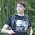# 2-D viscous, steady Burgers' equation: exact solution

Burgers' equation is a fluid mechanics fundamental partial differential equation which has the general 1-D form of:
\begin{equation} \frac{\partial u}{\partial t} + u \frac{\partial u}{\partial x} = \nu \frac{\partial^2 u}{\partial x^2}, \end{equation} in which, $u$ is velocity and $\nu$ is viscosity coefficient.

The exact and numerical solutions of Burgers' equation is thoroughly discussed in mathematical and CFD papers and books. But here, the exact solution of the 2-D, viscous, steady Burgers' equation will be explained. The results may be of interest to those who are going to validate the iterative solvers.

The steady, viscous, Burgers' equation is illustrated as follows:
\begin{equation} \boldsymbol{u}\cdot\boldsymbol{\nabla}\boldsymbol{u} = \nu \boldsymbol{\nabla}^2\boldsymbol{u}, \end{equation} which in Cartesian coordinate can be written as:
\begin{equation} \begin{cases} u\partial_{x}u+v\partial_{y}u = \nu \left(\partial_{xx}u^2+\partial_{yy}u^2\right) \\ u\partial_{x}v+v\partial_{y}v = \nu \left(\partial_{xx}v^2+\partial_{yy}v^2\right) \end{cases} \end{equation} and the conservation form is:
\begin{equation} \begin{cases} 0.5 \partial_{x}u^2+v\partial_{y}u = \nu \left(\partial_{xx}u^2+\partial_{yy}u^2\right) \\ u\partial_{x}v+0.5 \partial_{y}v^2 = \nu \left(\partial_{xx}v^2+\partial_{yy}v^2\right) \end{cases} \end{equation} Then using the following transformation:
\begin{equation} u = -\frac{2\nu}{\Phi} \frac{\partial \Phi}{\partial x}, \ \ v = -\frac{2\nu}{\Phi} \frac{\partial \Phi}{\partial y}, \end{equation} the system of equations will reduce to:
\begin{equation} \frac{\partial^2 \Phi}{\partial x^2} + \frac{\partial^2 \Phi}{\partial y^2} = 0. \end{equation} Subsequently, applying the separation approach $\Phi = f(x)g(y)$, the general solution would be:
\begin{equation} \Phi = a_1 + a_2x + a_3y + a_4xy + a_5\left[e^{\lambda(x-x_0)}+e^{-\lambda(x-x_0)}\right]\cos(\lambda y), \end{equation} in which constants $a_1$ to $a_5$ as well as $\lambda$ and $x_0$ are to be defined. As a result, the solution for velocity components are as follows:
\begin{equation} \begin{cases} u = -2\nu \left.\left[a_2 + a_4y + \lambda a_5 \left(e^{\lambda(x-x_0)}+e^{-\lambda(x-x_0)}\right)\cos(\lambda y)\right] \right/ \Phi \\ v = -2\nu \left.\left[a_3 + a_4x - \lambda a_5 \left(e^{\lambda(x-x_0)}+e^{-\lambda(x-x_0)}\right)\sin(\lambda y)\right] \right/ \Phi \end{cases} \end{equation} In the following, the exact solution is plotted for two different sets of constants:

# Case 1

In this case, the parameters are:
\begin{equation} a_1 = a_2 = 1.3\times10^{13}, a_3 = a_4 = 0, a_5 = 1, \lambda = 25, x_0 = 1, \nu = 0.04, \end{equation} and the solutions are illustrated in Fig. a.1 and a.2.

Fig. a.1: Case 1, solution for 2-D steady Burgers' equation, $u$.

Fig. a.2: Case 1, solution for 2-D steady Burgers' equation, $v$.

# Case 2

In this case, the parameters are:
\begin{equation} a_1 = a_2 = 110, a_3 = a_4 = 0, a_5 = 1, \lambda = 5, x_0 = 1, \nu = 0.1, \end{equation} and the solutions are illustrated in Fig. a.3 and a.4.

Fig. a.3: Case 2, solution for 2-D steady Burgers' equation, $u$.

Fig. a.4: Case 2, solution for 2-D steady Burgers' equation, $v$.

The numerical solution of the steady Burgers' equation applying Newton's method is discussed in the next sub-section.

The relevant Matlab code can be downloaded using the following link:
Matlab Code: Cartesian_2D_BURGER_Exact.m

#### 2 comments:

1.Very nice, but what about numerical techniques?

1.The numerical results will be published within a week. You are welcomed to follow the blog using the followers gadget to track the new topics.